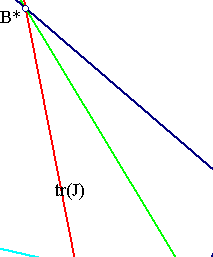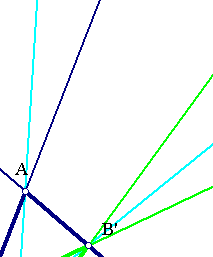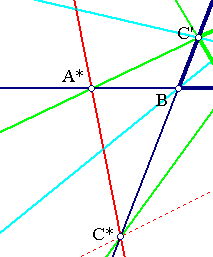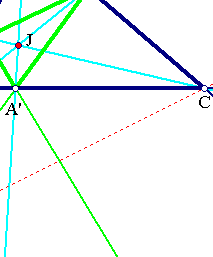##1. Trilinear Polar

Given the triangle of reference t = (ABC) and a point J, construct its Cevian triangle t' = (A'B'C'). Points {A',B',C'} are the traces of J on the sides of the triangle of reference ABC. The intersection points {A*,B*,C*} of corresponding side-pairs (AB, A'B'), (BC, B'C'), (CA, C'A') are collinear on a line tr(J) called trilinear polar of J with respect to t.A proof can be given by applying Desargues (see Desargues.html ) on triangles t, t' which are, per construction, point-perspective: J being the intersection point of the lines joining corresponding points {(A, A'), (B, B'), (C, C')}. Hence, by Desargues they are line-perspective: their corresponding side-lines intersect on a line tr(J).
Another proof can be given by considering the basic figure for harmonic conjugate points (see Harmonic.html ). In fact, consider the line of the two intersection points {A*,B*} and show that C* is also on that line. By the referred basic figure points {C',C*} are harmonic conjugate to {A, B}. Hence the bundle of four lines (CA,CB,CC',CC*) is a harmonic one and defines on line A*B* a quadruple of harmonic conjugate points. But line A'B' passes also (basic figure) from the harmonic conjugate of point C' with respect to {A,B}.
A third proof is given in (6) of ProjectiveBase.html .

##2. Trilinear Pole

Given the triangle of reference t = (ABC) and a line L the above procedure can be reversed and determine a point P whose trilinear polar is the given line L.
For this take the intersection points {A*,B*,C*} of L with the sides of the triangle and the corresponding harmonic conjugates {A',B',C'} with respect to point pairs {(B,C),(C,A),(A,B)}. Then lines {AA',BB',CC'} intersect at a point and this is the pole of L. The proof can be again based on Desargue's theorem. Sometimes I denote the pole P=tr-1(L) by the same symbol P=tr(L). In such cases it is usually clear from the context which symbols denote points and which lines.

##3. Some trilinear polars

 Trilinear polars are not defined for points coinciding with the vertices of the triangle of reference ABC. As limiting positions the sides of the triangle are trilinear polars of every point (except the vertices) on them.
 The most prominent trilinear polar is perhaps the line at infinity coinciding with the trilinear polar of the centroid of the triangle.
 Another interesting case is the symmedian point whose trilinear polar is the Lemoine axis of the triangle.
 Also the orthic axis should be mentioned in this respect. It is the trilinear polar of the orthocenter.
 It is easily seen that points on the medians of the triangle of reference have trilinear polars which are parallel to the sides to which the medians are drawn. Thus, they pass trhough the same point on the line at infinity determined by the side on which the median. Equivalently, the trilinear poles of lines parallel to one side of the triangle are on the median to that side. This is though an erroneous image. The truth is that the trilinear poles of lines passing through a fixed point are on a conic which passes through the three vertices of the triangle (a circumconic). Here the conic is a degenerate one consisting of two lines: the median and the corresponding side-line on which the median is drawn. For a picture of the general case look at IsotomicConicOfLine.html .
For more general conics resulting as sets of poles of parallel trilinear polars see Trilinear_Polar.html .

##4. Projective invariance

Trilinear polars/poles are projective invariants of pairs (ABC, P) consisting of a triangle and a point not lying on the side-lines of the triangle. For every two such pairs (A1B1C1,P1) and (A2B2C2,P2) there is a projectivity (f) mapping one pair onto the other in the sense f(A1)=A2, ..., f(P1)=P2. Since the constructions involved in the definition of trilinear polar are also projective invariant it follows that such a map transforms the trilinear polar of P1 with respect to A1B1C1 to the trilinear polar of P2 with respect to A2B2C2.
In particular, take (A0B0C0,G) to be a triangle and its centroid which has as trilinear polar tr(G)=L the line at infinity. It follows that the trilinear polar of every other pair (ABC,P) has trilinear pair tr(P) the image f(L) of the line at infinity, where (f) is the projectivity defined by the properties {f(A0)=A,f(B0)=B,f(C0)=C,f(G)=P}.

##5. The polar aspect

Consider an equilateral triangle and its center (A0B0C0,P0). The trilinear polar of P0 is the line at infinity. This line coincides also with the polar of P with respect to the circumcircle. This configuration can be generalized to a general pair (ABC,P) using a projectivity. By such a transformation the circumcircle of the equilateral maps to a conic (c) passing through the vertices of the triangle and the line at infinity maps to the trilinear polar tr(P) of P. Since the operation of constructing the polar with respect to a conic is also projectively invariant the trilinear polar tr(P) is also the polar of P with respect to the conic (c).

##6. Sequence of triangles

 Note that the trilinear polar of J with respect to triangle A'B'C' (called pre-cevian of ABC w.r. to J) is the same line tr(J). In fact, using the previous remarks, one can easily see that the trilinear polar of a triangle A'B'C' with respect to J is the same with the trilinear polar of the same point J with respect to the triangle with vertices the harmonic associates {A,B,C} of J with respect to A'B'C' (see Trilinears.html ).
Thus, taking harmonic associates of J with respect to A'B'C' and repeating the process we get infinite many triangles having the same trilinear polar with respect to the same point. The inverse procedure to create triangles with the same polar is to take the traces of J repeatedly. This creates a sequence of triangles converging to J.

 Fixing a triangle ABC, the relation between points and lines: point P <---> tr(P) of P, resembles the polarity defined by a conic. The similarity though is not far reaching. For example, taking a polar T(P) and the polars T(Q) for points Q on T(P), the trilinear polars T(Q) do not pass through a point (as the polars with respect to conics) but envelope a conic (this is discussed in TriangleConics.html ). Thus in antithesis with polarity with respect to a conic, taking trilinear polars with respect to a reference triangle ABC does not define a projective map. This is also seen from the expression of this map in any projective base {A,B,C,D} in which a point represented through aA+bB+cC has trilinear polar represented through the equation (1/a)x+(1/b)y+(1/c)z = 0.

##7. Perspectivity aspect

Triangles ABC and A'B'C' are point-perspective with respect to J. Hence, by Desargues theorem, they are also line perspective. From this perspectivity aspect the relation between the two triangles is symmetric. In fact, the center and the axis of perspectivity of two triangles are geometric constructs in which the two triangles, in general, participate symmetrically and indistinguishable the one from the other. In the special case of trilinear polar though this is not the case. Here we have a special perspectivity, by which the vertices of one triangle are on the sides of the other.
To variate on this point of view, one can define a perspectivity (see Perspectivity.html ) F by requiring from it to fix J and map {A,B,C} correspondingly to {A',B',C'}. This map and its inverse applied repeatedly to ABC produce the infinite sequence of triangles of section 6. The map is discussed in some extend in TrilinearProjectivity.html and the references given there.

##8. The two conics

There are two conics {c0,c1} related to a system (ABC, J) consisting of a triangle and a distinguished point not lying on its side-lines. Conic c0 is generated by the tripoles of all lines through J. Conic c1 is generated as envelope of all tripolars of points on the trilinear polar tr(J) of J.
Point J and line tr(J) are called respectively perspector and perspectrix of these conics. For a discussion of these conics it is convenient to introduce appropriate projective coordinates (see IsogonalGeneralized.html ). In the file TriangleConics.html is contained a discussion of the basic relations between these two conics.

Desargues.html
Harmonic.html
Harmonic_Bundle.html
IsogonalGeneralized.html
IsotomicConicOfLine.html
LineInTrilinears.html
Perspectivity.html
ProjectiveBase.html
TriangleConics.html
Trilinear_Polar.html
TrilinearProjectivity.html
Trilinears.html
Tripole.html